Home >> Radfords-cyclopedia-of-construction-vol-1-mechanical-drafting >> Architectural Drawings to Working Drawings 134 >> Pictorial Drawing 110_P1

Pictorial Drawing 110

isometric, fig, edges, shown, vertical, drawn and lines

Page: 1 2 3 4

PICTORIAL DRAWING 110. Graphical representations which give more or less of a picture effect, are sometimes very useful adjuncts to practical drafting.

To the untrained eye, such a drawing—of a building, for example—conveys a much clearer idea than plans and elevations. The same would be true of any other complicated structure. These pictorial drawings are of various kinds, as perspective, oblique projection, and isometric drawing. Of these three kinds, the first alone is the true picture drawing showing the actual appearance of any object as seen by the nat ural eye.

Unfortunately, however, the principles of perspective, while not difficult, are harder to acquire than those of oblique projection or isometric drawing, and the process of construct ing an accurate perspective drawing is longer and more tedious.

It is also true that in many eases the some what distorted isometric or oblique projections will supply to the mind all the pictorial assist ance that is necessary. The present section of this treatise will deal principally with isometric drawing and oblique projection.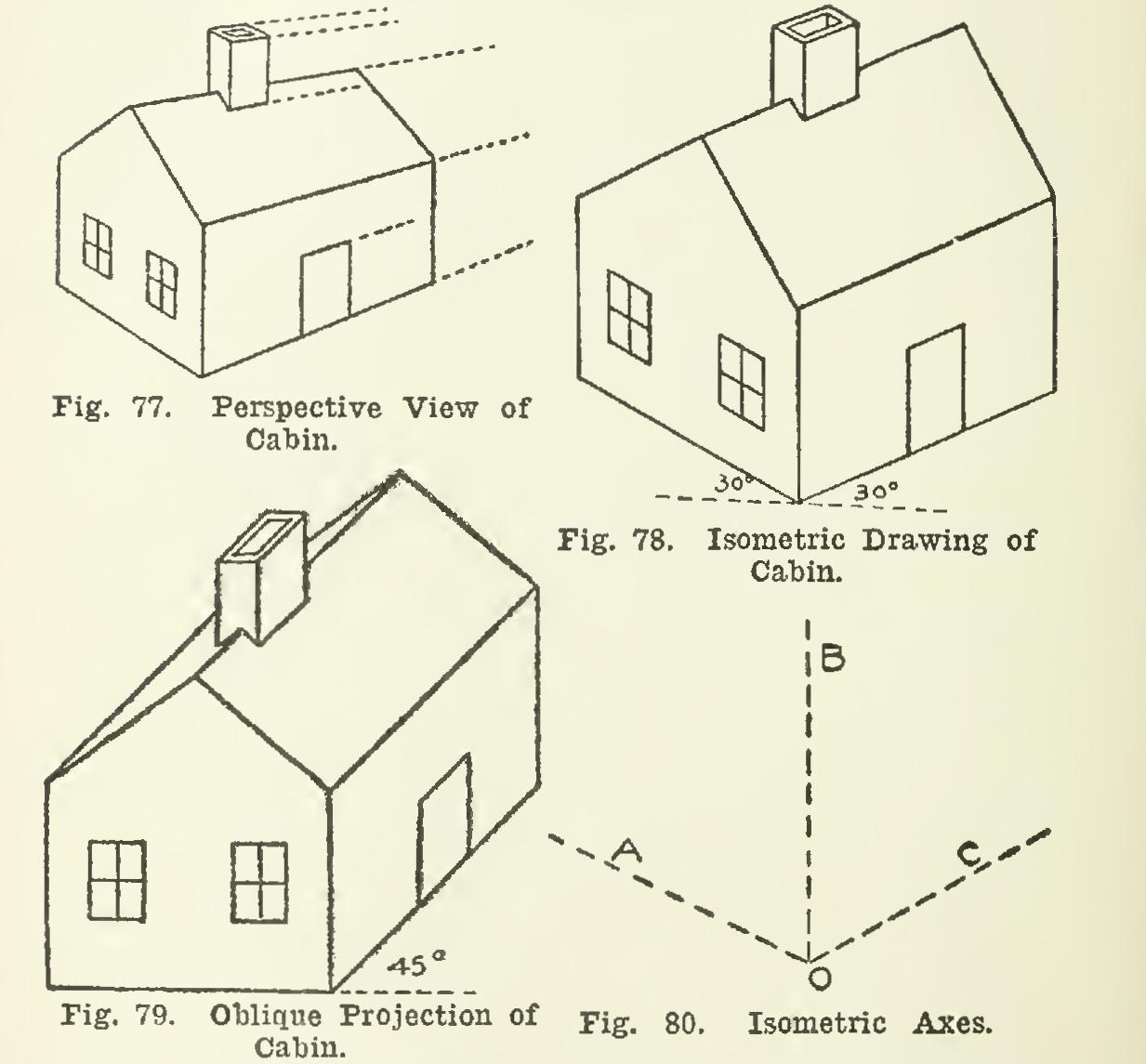111. For the sake of comparison, the same cabin has been shown in Figs. 77, 78, and 79, in the three different ways, Fig. 77 being a per spective view; Fig. 78, an isometric drawing; and Fig. 79, an oblique projection. In all three views, the vertical edges of the cabin are drawn as vertical lines, and therefore parallel.

In Figs. 7S and 79, all the other lines and edges which are actually parallel in space are drawn as parallel lines; but in the perspective of Fig. 77, the other lines which are really parallel in space are drawn as converging lines—as, for example,_the edges of the roof and the ridge, the top of the doorway, etc.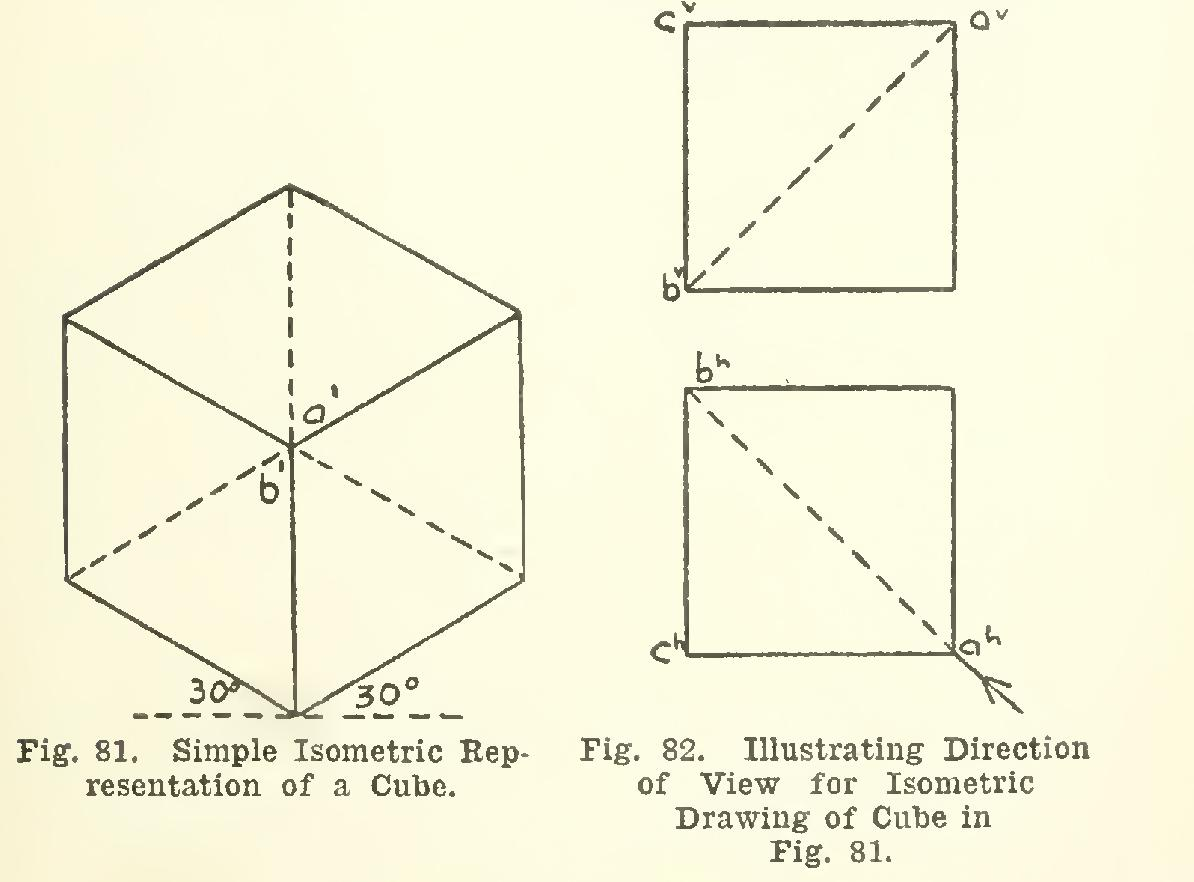Isometric Drawing 112. The principles of isometric drawing are best illustrated by reference, first, to a simple rectangular object as a cube or square prism. In such a solid there are three sets or systems of parallel edges; and in the simplest isometric drawing, these edges are drawn in three fixed directions, respectively parallel to what are called the isometric axes. These three axes are shown in Fig. 80, B vertical, and A and C at

30 degrees with the horizontal.

In any isometric drawing, lines parallel to the isometric axes are drawn in their true lengths.

113. The simplest isometric representation of a cube is shown in Fig. 81, except that ordi narily the dotted edges are omitted. All of the edges in this figure are full-length, and are either vertical or at 30 degrees with a hori zontal. The drawing represents the cube in the position of Fig. 82, with the view taken in the direction of the diagonal of the cube, as indi cated by the arrow. That that is the direction of the view, is shown in Fig. 81, from the fact that the lower back corner of the cube is directly behind the upper front corner a.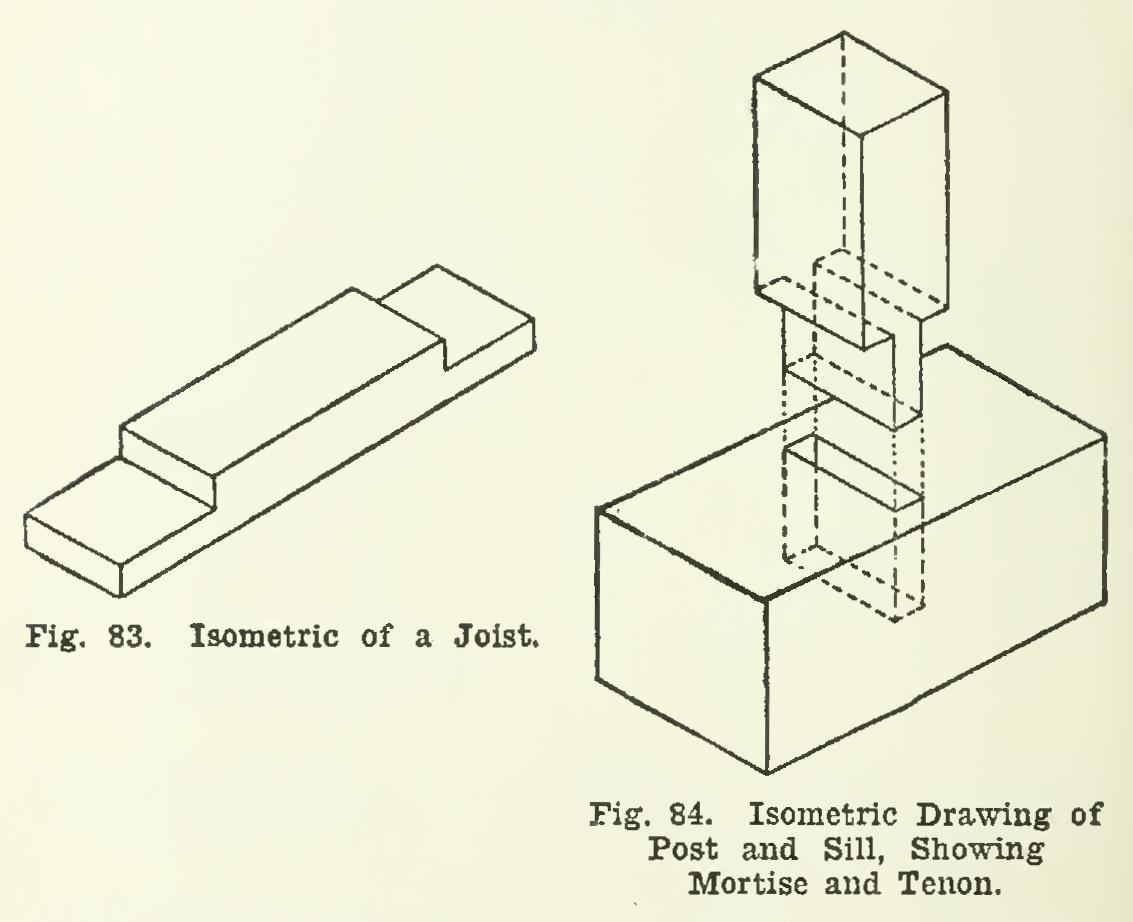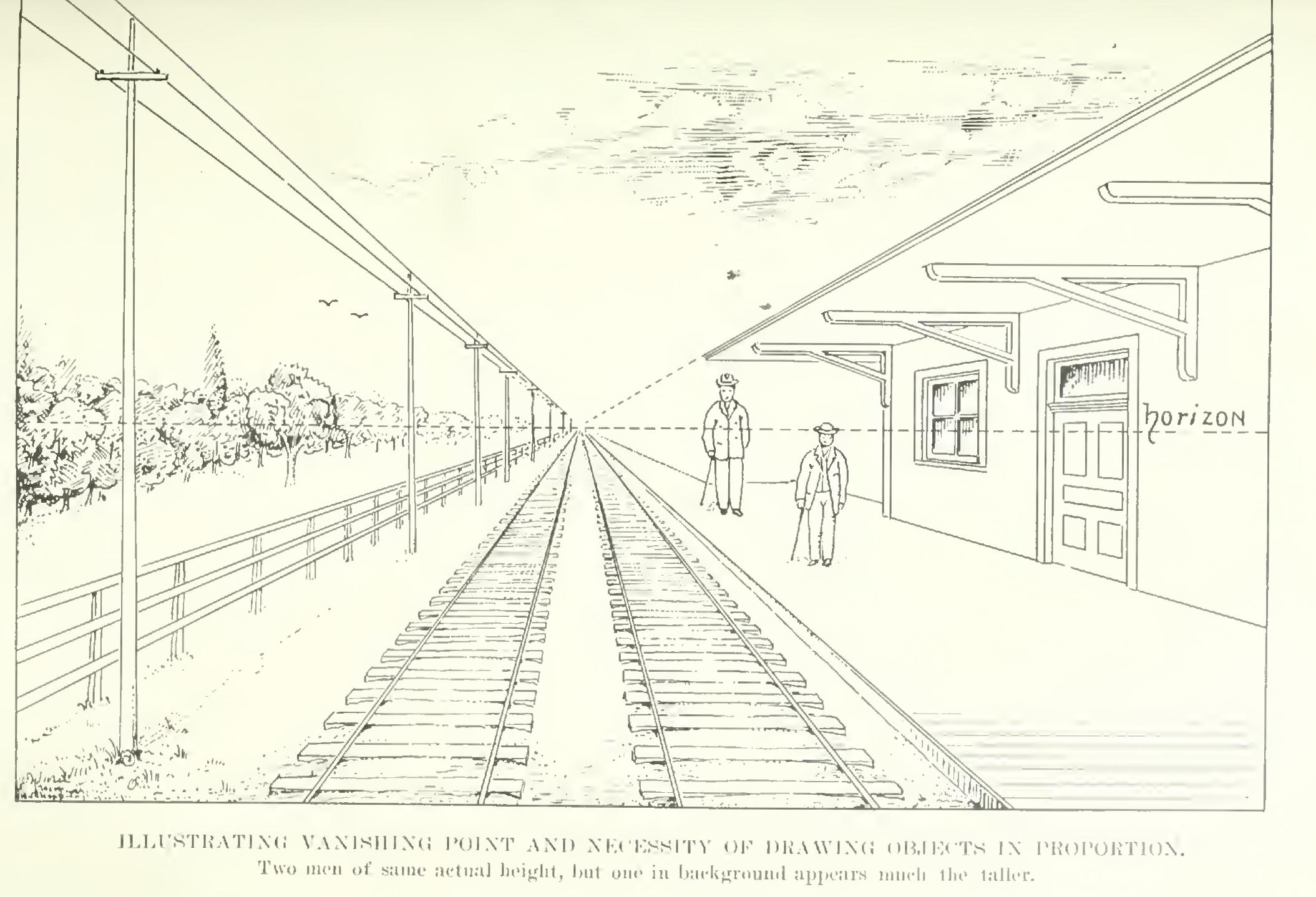The isometric view may be taken through either corner a or c, but always in the direction of a diagonal of the cube. Note that while the edges are shown in their true lengths, the angles are not shown in their true size.

Isometric drawing is especially appropriate for showing framing, mortises, tenons, etc.

114. A simple isometric is given in Fig. 83, where a piece of joist is shown ready for halving together with two other pieces.

115. The isometric of a post and sill, show ing a mortise and tenon, is drawn in Fig. 84.

116. To Draw an Isometric from a Given Plan and Elevation. Let A° and A', Fig. 85, be the elevation and plan of a wooden miter box, and let 0 be chosen for the lowest corner of the isometric. Then the horizontal edges of the box will be 30-degree lines in isometric; the vertical edges, vertical lines; and the real lengths will be shown (compare Figs. 81 and 82). Then, from 0' draw a 30-degree line to the right, equal to the length; one to the left, equal to the width; and one vertical, equal to the given height.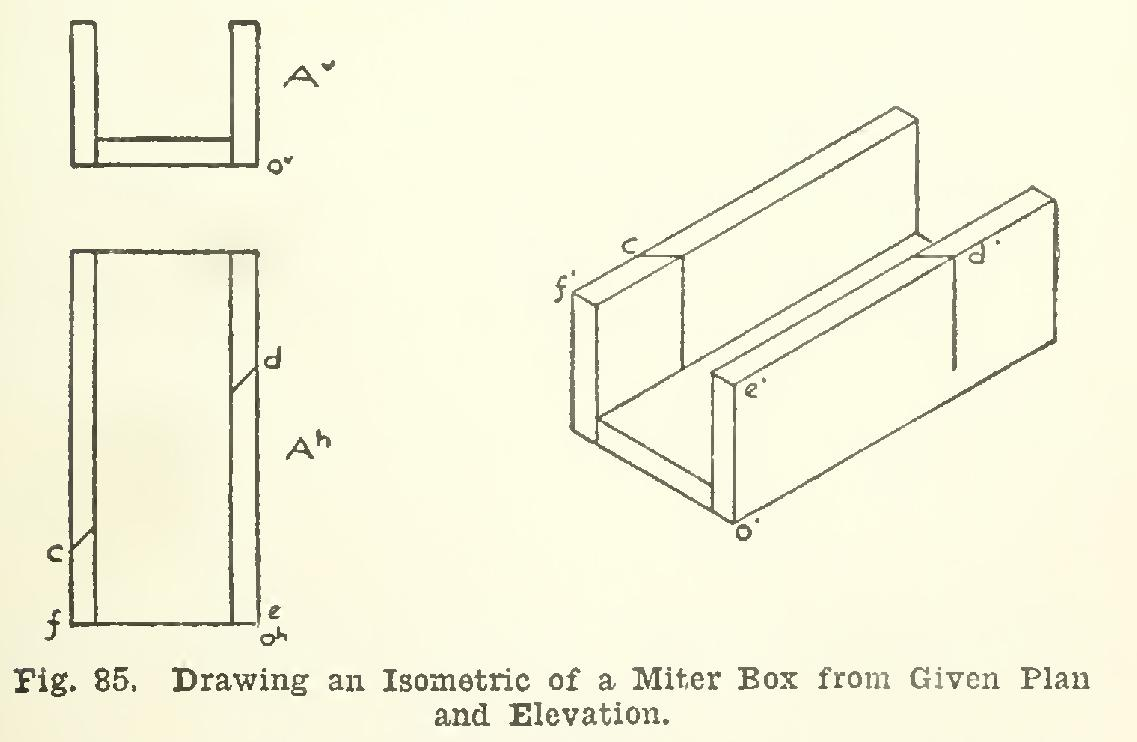The rest of the box may then be drawn, showing the thickness of the bottom and sides. The 45-degree saw-cut c-d, as shown in plan, is transferred to the isometric by simply laying off from f' and e' respectively the distances f-c and e-d, and joining c' and d' across the top of the box. The vertical cut showing on the sides of the box is then drawn in.

Page: 1 2 3 4